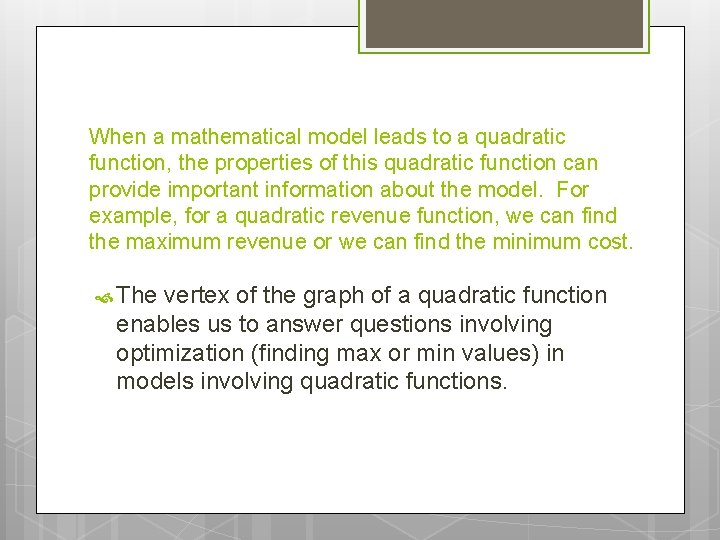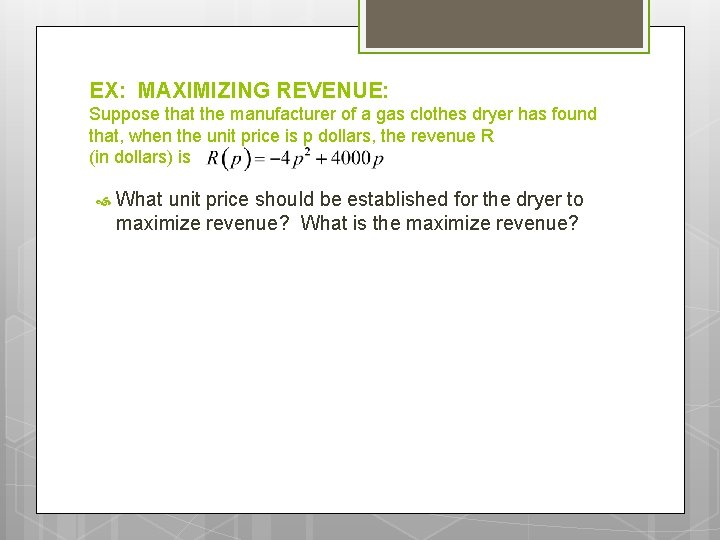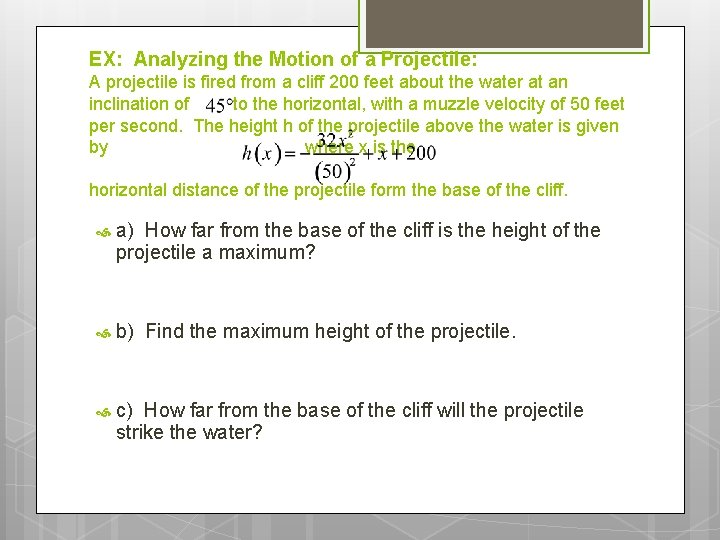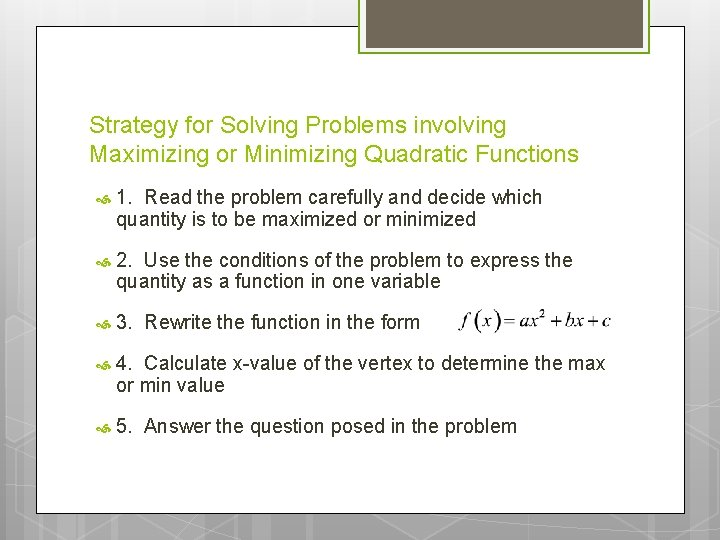# Quadratic Models Objectives Use the maximum or minimum

• Slides: 6Quadratic Models Objectives; Use the maximum or minimum value of a quadratic function to solve applied problemsWhen a mathematical model leads to a quadratic function, the properties of this quadratic function can provide important information about the model. For example, for a quadratic revenue function, we can find the maximum revenue or we can find the minimum cost. The vertex of the graph of a quadratic function enables us to answer questions involving optimization (finding max or min values) in models involving quadratic functions.EX: MAXIMIZING REVENUE: Suppose that the manufacturer of a gas clothes dryer has found that, when the unit price is p dollars, the revenue R (in dollars) is What unit price should be established for the dryer to maximize revenue? What is the maximize revenue?EX: Enclosing a Rectangular Field: David has available 400 yards of fencing and wishes to enclose a rectangular area. a) Express the area A of a rectangle as a function of the width w of the rectangle b) For what value of w is the area largest? c) What is the maximum area?EX: Analyzing the Motion of a Projectile: A projectile is fired from a cliff 200 feet about the water at an inclination of to the horizontal, with a muzzle velocity of 50 feet per second. The height h of the projectile above the water is given by where x is the horizontal distance of the projectile form the base of the cliff. a) How far from the base of the cliff is the height of the projectile a maximum? b) Find the maximum height of the projectile. c) How far from the base of the cliff will the projectile strike the water?Strategy for Solving Problems involving Maximizing or Minimizing Quadratic Functions 1. Read the problem carefully and decide which quantity is to be maximized or minimized 2. Use the conditions of the problem to express the quantity as a function in one variable 3. Rewrite the function in the form 4. Calculate x-value of the vertex to determine the max or min value 5. Answer the question posed in the problem